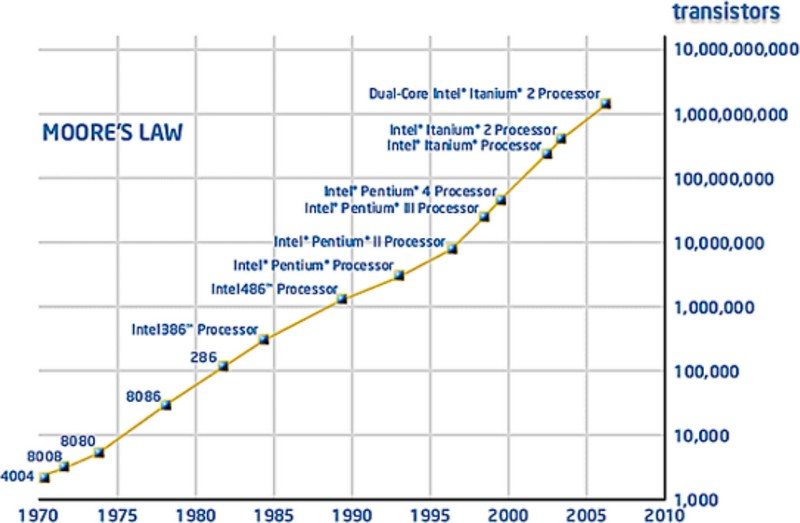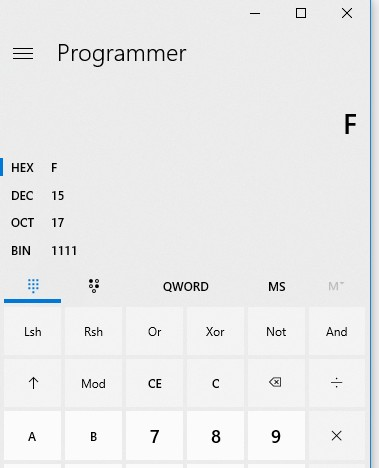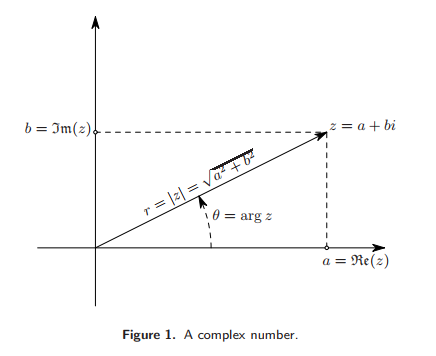# Number - (Numeral system|System of numeration|Representation of number)

A numeral system (or system of numeration) is a mathematical notation system for expressing numbers using digits (or other symbols). The numeral system gives the context that allows the (digits|symbols) to be interpreted.

A positional notation has the following properties:

Example, the symbols 11 is interpreted

For systems for classifying numbers according to their type, see Number System (Classification|Type).

## List

The Hindu–Arabic numeral system, base-10, is the most commonly used system in the world today for most calculations.

2 8 10 16
00000 0 0 0
00001 1 1 1
00010 2 2 2
00011 3 3 3
00100 4 4 4
00101 5 5 5
00110 6 6 6
00111 7 7 7
01000 10 8 8
01001 11 9 9
01010 12 10 A
01011 13 11 B
01100 14 12 C
01101 15 13 D
01110 16 14 E
01111 17 15 F
10000 20 16 10
10001 21 17 11
10010 22 18 12
10011 23 19 13
10100 24 20 14
10101 25 21 15

### Machine Data Representation

Most computers store data by block of bytes (of eight bits)

Representation of a full bytes 1111111 in:

 Base Note Representation 11111111 Eight Full Digit 377 Digits are not used completely (Not 777) 255 FF Digits are used completely

As the octal digit are not used completely, the Octal system is not the most practical number system to store a byte.

## Example

Python with an set expression in order to calculate the set of numbers that are made with:

• at-most-three-digit numbers.
base = 2
digits = {0, 1}
{(base**2)*x + base*y + z for x in digits for y in digits for z in digits}

{0, 1, 2, 3, 4, 5, 6, 7}


• at-most-four-digit numbers.
{(base**3)*w + (base**2)*x + base*y + z for x in digits for y in digits for z in digits for w in digits}

{0, 1, 2, 3, 4, 5, 6, 7, 8, 9, 10, 11, 12, 13, 14, 15}



## Documentation / Reference

Recommended PagesBit - Numeral system (Base-2) notation - Binary Number

The binary numeral system, or base-2 number system, represents numeric values using two symbols, 0 and 1. Owing to its straightforward implementation in digital electronic circuitry using logic gates,...Number - Babylonian numeral system, base-60

The sexagesimal numeral system or base-60, was the first positional system in the first language developed the Cuneiform It was used by astronomers Numeral System Parts Babylonian numerals and other...The radix or base is the number of unique digits, including zero, used to represent numbers in a positional numeral system. For the decimal system the radix is ten, because it uses ten digits: ...Number - Decimal (base 10) notation

Decimal is a numeral system with a base 10 because it has 10 characters (called digit): 0, 1, 2, 3, 4, 5, 6, 7, 8, 9 The usual decimal representation of whole numbers gives every...Number - Digit (Numeric symbol)

A digit is a numeric symbol of a numeral system. see The decimal notation (base 10) has 10 digits: 0,1,2,3,4,5,6,7,8,9. When used in combinations, they can represent more number. A whole field...Number - Field

A field is a collection of “numbers” with the operator: +, -, , / Different fields are like different classes obeying to the same interface. Same as ?? A field is also known as abody: corps...Number - Floating-point (system|notation) - (Float|Double) - Approximate numeric

The term floating point refers to the fact that the number's radix point can float, that is, it can be placed anywhere relative to the significant digits of the number. They are fractional numbers...Hexadecimal is a numeral system in base 16 (0,1,2,3,4,5,6,7,8,9,A,B,C,D,E,F). To see binary data in hexadecimal form, you use an hex editor. Hexadecimal is more compact to write than binary. For example,...
Number System (Classification|Type)

Numbers are classified according to how they are represented or according to the properties that they have. They have what's called a type. For systems for expressing numbers, see Numeral system. ...Number System - Complex Number -

A real number plus an imaginary number is a complex number. Complex numbers are a number system. A complex number has a real part and an imaginary part. where: i is an imaginary number Complex...